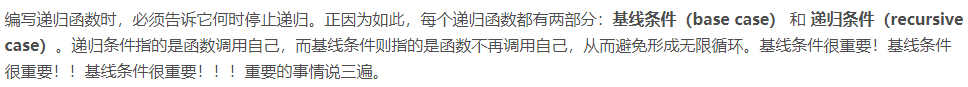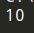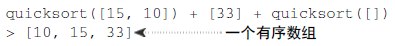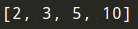# 《算法图解》学习笔记（四）：分而治之和快速排序（附代码）

## 一、分而治之

《算法图解》学习笔记（三）：递归和栈（附代码） 深入介绍了递归。我们将探索 分而治之（divide and conquer，D&C） —— 一种著名的递归式问题解决方法。只能解决一种问题的算法毕竟用处有限，而D&C提供了解决问题的思路，是另一个可供使用的工具。面对新问题时，你不再束手无策，而是自问：“使用分而治之能解决吗？”

D&C好像很不错，那我们就一直用这个方法好了，然而它并不那么容易掌握，先来看示例。

#### 1）例子一1. 找出基线条件，这种条件必须尽可能简单。
2. 不断将问题分解（或者说缩小规模），直到符合基线条件。1. 找出简单的基线条件；
2. 确定如何缩小问题的规模，使其符合基线条件。

D&C并非可用于解决问题的算法，而是一种解决问题的思路。

#### 2）例子二def sum(arr):
total = 0
for x in arr:
total += x

print(sum([1, 2, 3, 4]))## 二、快速排序def quicksort(array):
if len(array) < 2:
return array• 一个由所有小于基准值的数字组成的子数组；
• 基准值；
• 一个由所有大于基准值的数组组成的子数组。1. 选择基准值。
2. 将数组分成两个子数组：小于基准值的元素和大于基准值的元素。
3. 对这两个子数组进行快速排序。python版本代码如下：

def quicksort(array):
if len(array) < 2:
#基本情况下，具有0或1个元素的数组是已经“排序”的
return array
else:
#递归情况
pivot = array
#小于基准值的所有元素的子数组
less = [i for i in array[1:] if i <= pivot]
#大于基准值的所有元素的子数组
greater = [i for i in array[1:] if i > pivot]
return quicksort(less) + [pivot] + quicksort(greater)

print(quicksort([10, 5, 2, 3]))c++版本代码如下：

#include <iostream>
#include <vector>

using std::cout;
using std::endl;

template <typename T>
std::vector<T> quicksort(const std::vector<T>& arr) {
// base case, arrays with 0 or 1 element are already "sorted"
if (arr.size() < 2)
return arr;

// recursive case
const T* pivot = &arr.front() + arr.size() / 2 - 1; // set the pivot somewhere in the middle
std::vector<T> less;  // vector to store all the elements less than the pivot
std::vector<T> greater;  // vector to store all the elements greater than the pivot

for (const T* item = &arr.front(); item <= &arr.back(); item++) {
if (item == pivot) continue; // skip pivot element
if (*item <= *pivot) less.push_back(*item);
else greater.push_back(*item);
}

std::vector<T> sorted_less = quicksort(less);
std::vector<T> sorted_greater = quicksort(greater);
// concatenate less part, pivot and greater part
sorted_less.push_back(*pivot);
sorted_less.insert(sorted_less.end(), sorted_greater.begin(), sorted_greater.end());

return sorted_less;
}

int main() {
std::vector<int> arr = {10, 5, 2, 3};
std::vector<int> sorted = quicksort(arr);
for (int num : sorted) {
cout << num << " ";
}
cout << endl;
}


## 三、再谈大O 表示法• 这里说的最糟情况和平均情况是什么意思呢？
• 若快速排序在平均情况下的运行时间为O(n log n)，而合并排序的运行时间总是O(n log n)，为何不使用合并排序？它不是更快吗？

#### 1）比较合并排序和快速排序

def print_items(list):
for item in list:
print(item)


from time import sleep
def print_items2(list):
for item in list:
sleep(1)
print(item)c 是算法所需的固定时间量，被称为常量。例如，print_ items 所需的时间可能是10毫秒 * n，而 print_items2 所需的时间为1秒 * n#### 2）平均情况和最糟情况## 四、总结

• D&C将问题逐步分解。使用D&C处理列表时，基线条件很可能是空数组或只包含一个元素的数组。
• 实现快速排序时，请随机地选择用作基准值的元素。快速排序的平均运行时间为O(n log n)。
• 大O表示法中的常量有时候事关重大，这就是快速排序比合并排序快的原因所在。
• 比较简单查找和二分查找时，常量几乎无关紧要，因为列表很长时，O(log n)的速度比O(n)快得多。

## 参考文章